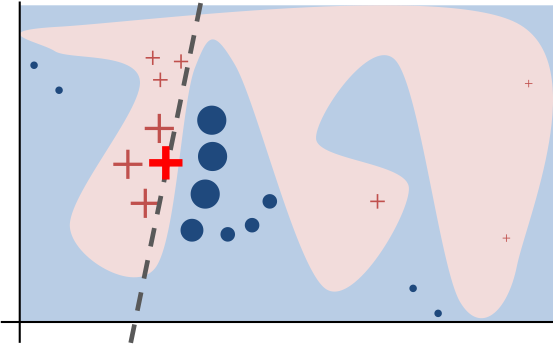# LIME: Local Interpretable Model-agnostic Explanations

The type of explanation LIME offers is a surrogate model that approximates a black box prediction locally. The surrogate model is a sparse linear model, which means that the surrogate model is interpretable (in this case, it’s weights are meaningful). This simpler model can thus help to explain the black box prediction, assuming the local approximation is actually sufficiently representative.

The intuition behind this is provided in the README:

Intuitively, an explanation is a local linear approximation of the model’s behaviour. While the model may be very complex globally, it is easier to approximate it around the vicinity of a particular instance. While treating the model as a black box, we perturb the instance we want to explain and learn a sparse linear model around it, as an explanation. The figure below illustrates the intuition for this procedure. The model’s decision function is represented by the blue/pink background, and is clearly nonlinear. The bright red cross is the instance being explained (let’s call it X). We sample instances around X, and weight them according to their proximity to X (weight here is indicated by size). We then learn a linear model (dashed line) that approximates the model well in the vicinity of X, but not necessarily globally. (README)Having a global approximation of a black box classifier would mean that we can have a white box classifier that is able to accurately approximate the black box predictions for any input. If we would have such a white box model, we would have probably used that model instead of the black box. Locally, i.e. in the more limited feature range of a single input, white box classifiers can more reliably approximate the black box predictions. Notice that the local surrogate does not predict the true labels, but the labels as predicted by the black box model.

The authors of LIME also wrote an accessible blog post explaining LIME.